# Elliptic Integral

Also found in: Dictionary, Wikipedia.
Related to Elliptic Integral: elliptic integral of the second kind

## elliptic integral

[ə′lip·tik ′int·ə·grəl]
(mathematics)
An integral over x whose integrand is a rational function of x and the square root of p (x), where p (x) is a third- or fourth-degree polynomial without multiple roots.
McGraw-Hill Dictionary of Scientific & Technical Terms, 6E, Copyright © 2003 by The McGraw-Hill Companies, Inc.
The following article is from The Great Soviet Encyclopedia (1979). It might be outdated or ideologically biased.

## Elliptic Integral

any integral of the type

R (x, y) dx

where R(x, y) is a rational function of x andbeing a third-or fourth-degree polynomial without multiple roots.

The integral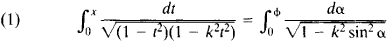is called an incomplete elliptic integral of the first kind, and the integral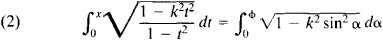is called an incomplete elliptic integral of the second kind. Here, k is the modulus of the elliptic integral, 0 < k < 1 (x = sin φ, t = sin α). The integrals on the left-hand side of equations (1) and (2) are known as Jacobi’s normal forms, and the integrals on the right-hand side are known as Legendre’s normal forms. When x = 1 or φ = π/2, the elliptic integrals are said to be complete and are designated by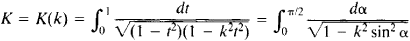and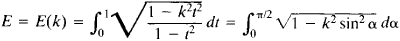respectively.

Elliptic integrals owe their name to their appearance in the problem of calculating the length of an arc of an ellipse ua sin α v = b cos α (a > b). The length of an arc of the ellipse is expressed by the formula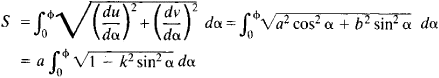where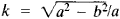is the eccentricity of the ellipse. The length of one-fourth of the circumference of an ellipse is equal to E(k). The inverse functions of elliptic integrals are called elliptic functions.

References in periodicals archive ?
k is the complete elliptic integral of the second kind of modulus k and additional modulus of complete elliptic integrals, k' = [square root of 1 - [k.sup.2]] .
where E([THETA] | m) is the elliptic integral of the second kind, am(x | m) is the Jacobi elliptic function amplitude, and E(m) is the complete elliptic integral of the second kind.
where F, E, and [PI] are the first, second, and third kind of complete elliptic integral, respectively, in which K = [square root of ((4[bar.r] x [bar.[rho]]) / ([([bar.r] + [bar.[rho]]).sup.2] + [[bar.z].sup.2.sub.p]))] and [n.sub.1] = (-4[bar.[rho]] x [bar.r])/[([bar.[rho]] + [bar.r]).sup.2].
A calculation of [partial derivative][u.sup.*.sub.ax]/[partial derivative]n, with the use of the relation for K'(m) in terms of the complete elliptic integral of the second kind E(m), gives
The parameter k is the elliptic modulus or eccentricity that must satisfy 0 < [k.sup.2] < 1 and < is called the argument of the normal elliptic integral and is usually taken to be 0 < [phi] < [pi]/2.
For a generic value of t, the integral is said to be incompletely expressed by the elliptic integral of first kind as
Incomplete elliptic integral of the third kind [PI] is defined as
Note that all functions used in the analysis (e.g., elliptic integrals of first kind) are available in most mathematical software packages.
Assume that sn(x, k), cn(x, k), dn(x, k) are the Jacobian elliptic functions with the modulus k, K(k) is the first kind of complete elliptic integral, E([phi], k) is the normal elliptic integral of the 2nd kind, and [PI]([phi], [[alpha].sup.2], k) is the normal elliptic integral of the 3rd kind .
where [K.sub.j] is the complete elliptic integral of the first kind, and cd is Jacobi elliptic function.

Site: Follow: Share:
Open / Close